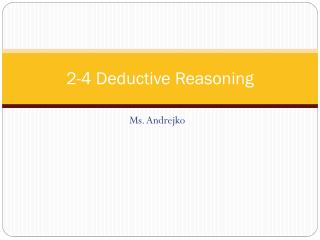# 2-4 Deductive Reasoning - PowerPoint PPT PresentationDownload Presentation2-4 Deductive Reasoning

2-4 Deductive ReasoningDownload Presentation## 2-4 Deductive Reasoning

- - - - - - - - - - - - - - - - - - - - - - - - - - - E N D - - - - - - - - - - - - - - - - - - - - - - - - - - -
##### Presentation Transcript

1. 2-4 Deductive Reasoning Ms. Andrejko

2. Real World

3. Vocabulary • Deductive reasoning- uses facts, rules, definitions, or properties to reach logical conclusions from given statements • Unlike inductive reasoning which uses patterns/observations • Law of Detachment- if pq is a true conditional, and p is true, then q is is also true • Law of Syllogism- if pq and qr are true statements, then pr is a true statement • Note: Stating a counterexample is not a valid method of proving a conjecture.

4. Laws • Detachment: • If a car is out of gas, then it will not start. Sarah’s car is out of gas. • Valid conclusion: Sarah’s car will not start. • Syllogism: • If you get a job, then you will earn money. If you earn money, then you will buy a car. • Valid conclusion: If you get a job, then you will buy a car.

5. Examples – Inductive or deductive? • 1. A dental assistant notices a patient has never been on time for an appointment, he concludes the patient will be late for her next appointment. • 2. If Eduardo decides to go to a concert tonight, he will miss the football game. Tonight Eduardo went to a concert. Eduardo missed football practice. Inductive Deductive

6. Practice– Inductive or deductive? • 1. A person must be insured in order to drive a car. Andy is driving a car. Andy is insured. • 2. Whenever Julie attends a tutoring session she notices that her grades have improved. Julia attends a tutoring session and she concludes that her grades will improve. Deductive Inductive

7. Examples – Valid or Invalid? • Given: If the sum of the measures of two angles is 180, then the angles are supplementary. m∠A + m∠B is 180. Conclusion: ∠A and ∠B are supplementary. 2. Given: If the sum of the measures of two angles is 90, then the angles are complementary. m∠ABC is 45 and m∠DEF is 48. Conclusion: ∠ABC and ∠DEF are complementary. VALID – Law of detachment INVALID: 45 + 48 = 93 , 93 ≠ 90.

8. Practice– Valid or Invalid? • Given: If a point is the midpoint of a segment, then it divides the segment into two congruentsegments. R is the midpoint of Conclusion: 2. Given: If 3 points are noncollinear, they determine a plane. Points A,B, and C, lie in plane G. Conclusion: Points A, B, and C are noncollinear. VALID – Law of detachment INVALID: points A, B, and C, could be collinear and form a line.

9. Examples- Law of Syllogism • If two angles are complementary, then the sum of their measures is 90.If the sum of the measures of two angles is 90, then both of the angles are acute. • If the heat wave continues, then air conditioning will be used more frequently. If air conditioning is used more frequently, then energy costs will be higher. If 2 angles are complementary, then both of the angles are acute. If the heat wave continues, then energy costs will be higher.

10. Examples- Law of Syllogism • 3. If it is Tuesday, then Marla tutors chemistry. If Marla tutors chemistry, then she arrives home at 4 P.M. • 4. If two lines are perpendicular, then they intersect to form right angles. Lines r and s form right angles. • 5. If two angles form a linear pair, then the two angles are supplementary. If two angles are supplementary, then the sum of their measures is 180. If it’s a Tuesday, then Marla arrives home at 4. NO VALID CONCLUSION If 2 angles form a linear pair, then the sum of their measures is 180.

11. FOLDABLE LOGIC TAB Law of Detachment Law of syllogism